# yihee

childe7
Mar. 31, 2023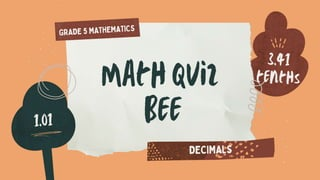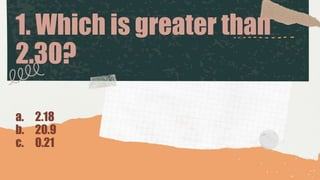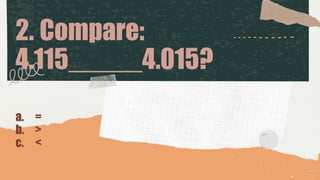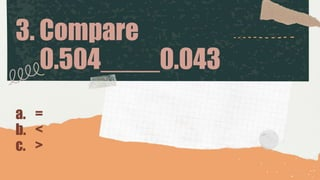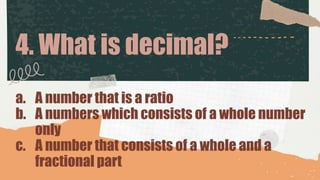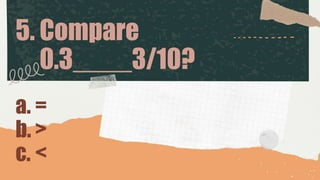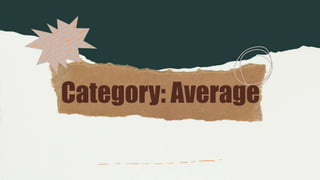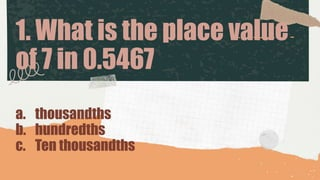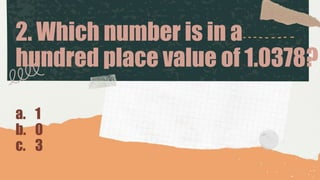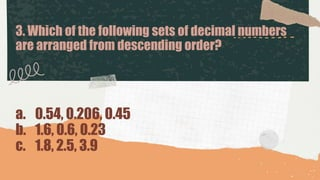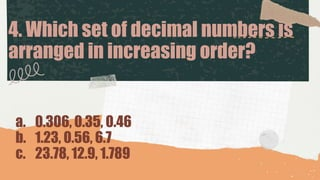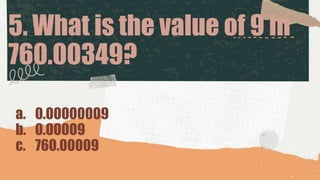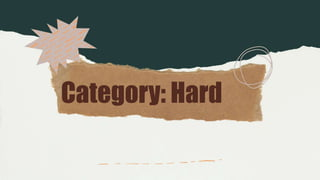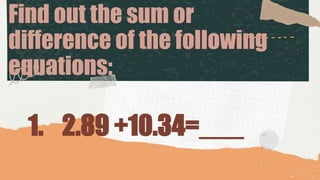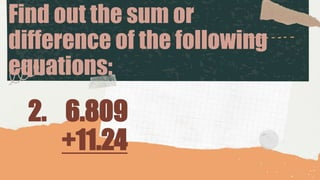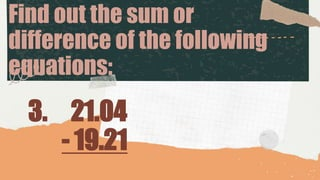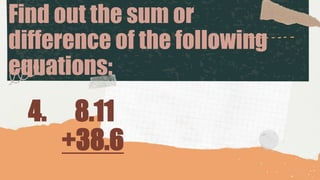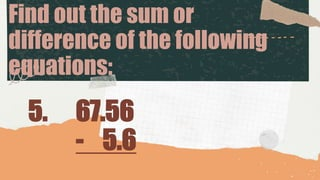1 of 19

### yihee

1. jjj
2. Category: Easy
3. 1. Which is greater than 2.30? a. 2.18 b. 20.9 c. 0.21
4. 2. Compare: 4.115_____4.015? a. = b. > c. <
5. 3. Compare 0.504____0.043 a. = b. < c. >
6. 4. What is decimal? a. A number that is a ratio b. A numbers which consists of a whole number only c. A number that consists of a whole and a fractional part
7. 5. Compare 0.3____3/10? a. = b. > c. <
8. Category: Average
9. 1. What is the place value of 7 in 0.5467 a. thousandths b. hundredths c. Ten thousandths
10. 2. Which number is in a hundred place value of 1.0378? a. 1 b. 0 c. 3
11. 3. Which of the following sets of decimal numbers are arranged from descending order? a. 0.54, 0.206, 0.45 b. 1.6, 0.6, 0.23 c. 1.8, 2.5, 3.9
12. 4. Which set of decimal numbers is arranged in increasing order? a. 0.306, 0.35, 0.46 b. 1.23, 0.56, 6.7 c. 23.78, 12.9, 1.789
13. 5. What is the value of 9 in 760.00349? a. 0.00000009 b. 0.00009 c. 760.00009
14. Category: Hard
15. Find out the sum or difference of the following equations: 1. 2.89 +10.34=___
16. Find out the sum or difference of the following equations: 2. 6.809 +11.24
17. Find out the sum or difference of the following equations: 3. 21.04 - 19.21
18. Find out the sum or difference of the following equations: 4. 8.11 +38.6
19. Find out the sum or difference of the following equations: 5. 67.56 - 5.6